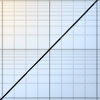# Resources tagged with: Exponential function

Filter by: Content type:
Age range:
Challenge level:

### There are 5 results

Broad Topics > Functions and Graphs > Exponential function### Gosh Cosh

##### Age 16 to 18 Challenge Level:

Explore the hyperbolic functions sinh and cosh using what you know about the exponential function.### Integral Equation

##### Age 16 to 18 Challenge Level:

Solve this integral equation.### Exponential Trend

##### Age 16 to 18 Challenge Level:

Find all the turning points of y=x^{1/x} for x>0 and decide whether each is a maximum or minimum. Give a sketch of the graph.### Hyperbolic Thinking

##### Age 16 to 18 Challenge Level:

Explore the properties of these two fascinating functions using trigonometry as a guide.### What Are Complex Numbers?

##### Age 16 to 18

This article introduces complex numbers, brings together into one bigger 'picture' some closely related elementary ideas like vectors and the exponential and trigonometric functions and. . . .